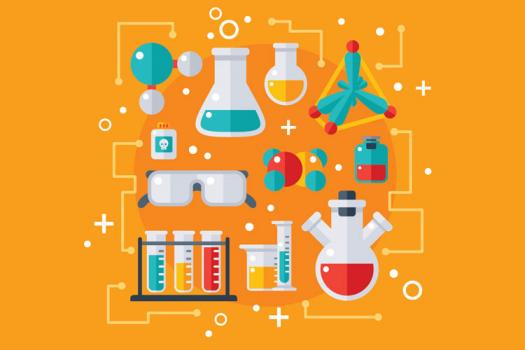# Take This 10th Grade Chemistry Quiz

10 Questions | Total Attempts: 106SettingsWe all know that Chemistry books can sometimes be very tiresome and we’re going to save you from them. We are your knights in shining armor, here to sweep you away from those sleep-inducing textbooks and show you a new world of revision. Take this quiz now.

Related Topics
• 1.
What is the relative formula mass of copper sulfate CuSO4?
• A.

160 amu

• B.

96 amu

• C.

128 amu

• D.

144 amu

• 2.
What is the relative formula mass of MgF2?
• A.

60 amu

• B.

62 amu

• C.

43 amu

• D.

64 amu

• 3.
Ammonium nitrate NH4NO3 is used as a fertiliser. What is the % mass of nitrogen in it?
• A.

21%

• B.

37%

• C.

17.5%

• D.

35%

• 4.
A reaction that could theoretically produce 200g of product only produces 140g. What is its % yield?
• A.

70%

• B.

14.2%

• C.

142%

• D.

7%

• 5.
A compound contains 13.5g of calcium, 10.8g of oxygen and 0.675g of hydrogen. What is its empirical formula?
• A.

CaOH

• B.

CaOH2

• C.

CaO2H

• D.

Ca(OH)2

• 6.
If 9g of aluminium reacts with 35.5g of chlorine, what is the empirical formula of the compound formed?
• A.

AlCl

• B.

AlCl2

• C.

AlCl3

• D.

Al3Cl

• 7.
Sodium hydroxide reacts with chlorine to make bleach as shown by this reaction. 2NaOH + Cl2 → NaOCl + NaCl + H2O. If we have a solution containing 100g of sodium hydroxide, how much chlorine gas should we pass through the solution to make bleach?
• A.

28.65g

• B.

82.45g

• C.

88.75g

• D.

86.57g

• 8.
Calcium reacts with oxygen as shown by this reaction 2Ca + O2 → 2CaO. What mass of oxygen will react with 60g of calcium?
• A.

12g

• B.

24g

• C.

6g

• D.

14g

• 9.
What is the % mass of hydrogen in methane CH4?
• A.

20%

• B.

25%

• C.

75%

• D.

12.5%

• 10.
What % mass of magnesium oxide is magnesium?
• A.

60%

• B.

40%

• C.

30%

• D.

70%